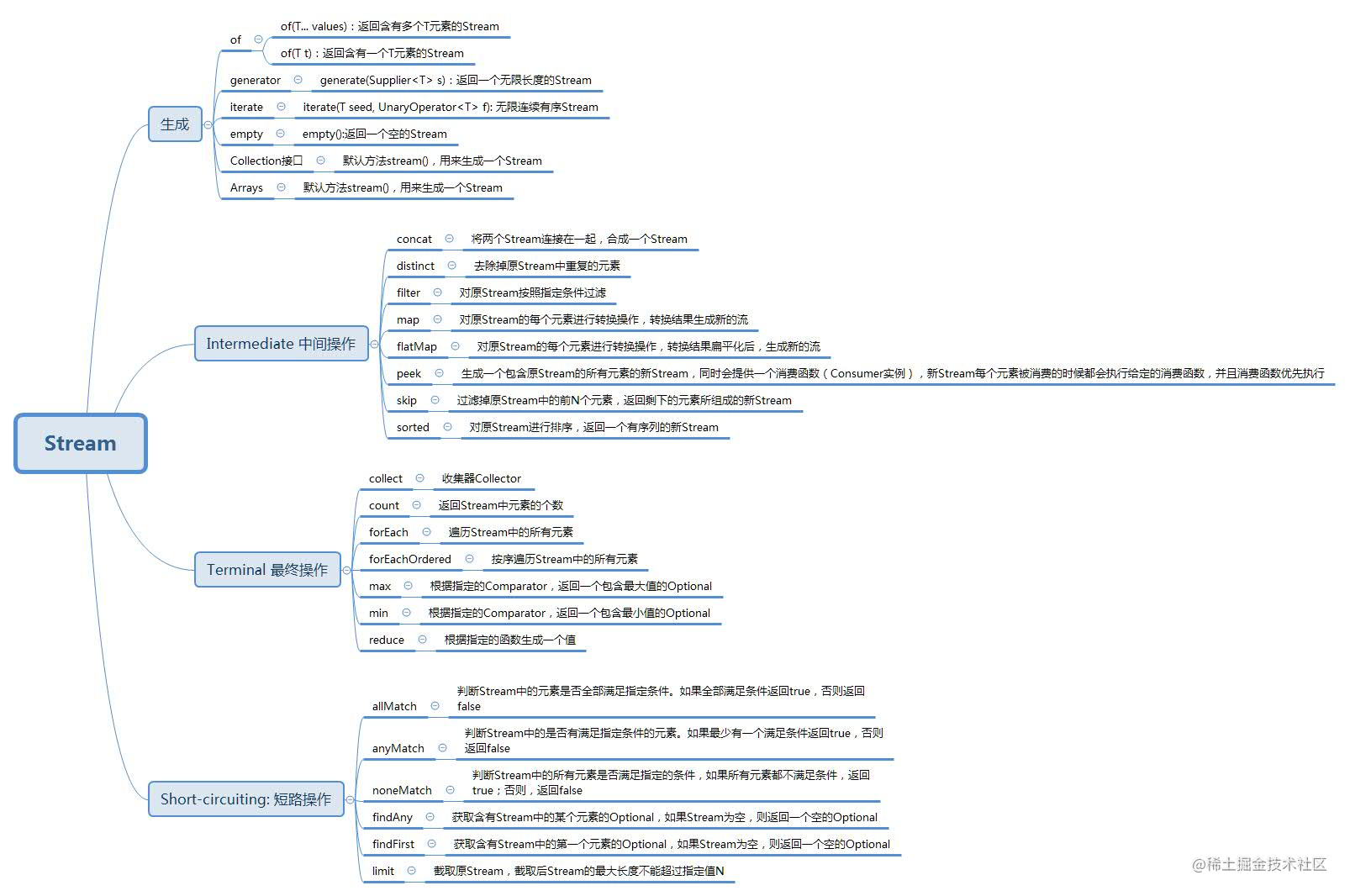# 精讲stream，万物皆可stream

## 引言

``````public long getCountsOfMaleStudent(List<Student> students) {
long count = 0;
for (Student student : students) {
if (student.isMale()) {
count++;
}
}
return count;
}

``````public long getCountsOfMaleStudent(List<Student> students) {
return students.stream().filter(Student::isMale).count();
}

• 回归问题本质，按照心智模型思考问题。
• 延迟加载。
• 简化代码。

## 创建流

``````List<Integer> numbers = Arrays.asList(1, 2, 3);
Stream<Integer> numberStream = numbers.stream();

String[] words = new String[]{"one", "two"};
Stream<String> wordsStream = Arrays.stream(words);

``````// 创建流
Stream<Integer> numbers = Stream.of(1, 2, 3);
// 创建空流
Stream<String> emptyStream = Stream.empty();
// 创建一个元素为“hi”的无限流
Stream<String> infiniteString = Stream.generate(() -> "hi");
// 创建一个从0开始的递增无限流
Stream<BigInteger> integers = Stream.iterate(BigInteger.ZERO, n -> n.add(BigInteger.ONE));

``````Stream<Double> top10 = Stream.generate(Math::random).limit(10);

``````// 3, 4
Stream<Integer> skipedStream = Stream.of(1, 2, 3, 4).skip(2);
// hello,world
Stream<String> concatedStream = Stream.concat(Stream.of("hello"), Stream.of(",world"));

## 常用的流操作

### filter

filter()方法的作用就是根据输入的条件表达式过滤元素。

``````Stream<T> filter(Predicate<? super T> predicate);

``````Stream.of("a", "1b", "c", "0x").filter(value -> isDigit(value.charAt(0)));

1b, 0x

### map

map()的主要作用是通过映射函数转换成新的数据。 接口定义如下：

``````<R> Stream<R> map(Function<? super T, ? extends R> mapper);

``````Stream.of("a", "b", "c").map(String::toUpperCase);

A, B, C

### flatMap

flatMap()的作用类似于map()，但它通过Function返回的依然是一个Stream，也即是把多个Stream转换成一个扁平的Stream。 接口定义如下：

``````<R> Stream<R> flatMap(Function<? super T, ? extends Stream<? extends R>> mapper);

``````Stream<List<Integer>> listStream = Stream.of(asList(1, 2), asList(3, 4));
Stream<Integer> integerStream = listStream.flatMap(numbers -> numbers.stream());

[1, 2, 3, 4]

## 有状态的转换

### distinct

distinct()的主要作用是去除流中的重复元素。和Oracle的distinct一个作用。 举例如下：

``````Stream<String> distinctStream = Stream.of("one", "one", "two", "three").distinct();

one, two, three

### sorted

sorted()的主要作用是对流按照指定的条件进行排序。 接口定义如下：

``````Stream<T> sorted(Comparator<? super T> comparator);

``````Stream<String> sortedStream = Stream.of("one", "two","three").sorted(Comparator.comparing(String::length).reversed());

[three, one, two]

## Optional类型

``````// 生成了一个Optional数据
Optional<String> maxStrOpt = Stream.of("one", "two", "three").max(String::compareToIgnoreCase);
// 如果值存在的情况下，把数据添加到List中
// 把结果映射为大写，然后取出。
Optional<String> upperResult = maxStrOpt.map(String::toUpperCase);System.out.println(upperResult.get());
// 值为空的情况下的后续处理
maxStrOpt.orElse("");
// 添加默认值""
maxStrOpt.orElseGet(() -> System.getProperty("user.dir"));
// 通过表达式返回结果
maxStrOpt.orElseThrow(RuntimeException::new); // 抛出异常

## 聚合操作

### count

count()的作用是统计元素的总数，很多时候需要配合filter一起使用。 一个例子：

``````long count = Stream.of("one", "two", "three").filter(word->word.contains("o")).count();

2

### max/min

max/min()的主要作用是取得元素的最大值/最小值。 接口定义如下：

``````Optional<T> max(Comparator<? super T> comparator);
Optional<T> min(Comparator<? super T> comparator);

``````Optional<String> maxStrOpt = Stream.of("one", "two", "three").max(String::compareToIgnoreCase);System.out.println(maxStrOpt.get());

two

### findFirst/findAny

`findFirst()`的主要作用是找到第一个匹配的结果。 `findAny()`的主要作用是找到找到任意匹配的一个结果。它在并行流中特别有效，因为只要在任何分片上找到一个匹配元素，整个计算就会结束。

``````Optional<T> findFirst();
Optional<T> findAny();

``````Optional<String> findFirstResult = Stream.of("one", "two", "three").filter(word -> word.contains("o").findFirst();
System.out.println(findFirstResult.get());
Optional<String> findAnyResult = Stream.of("one", "two", "three").filter(word -> word.contains("t").findAny();
System.out.println(findAnyResult.get());

one two

### anyMatch/allMatch/noneMatch

``````boolean anyMatch(Predicate<? super T> predicate);
boolean allMatch(Predicate<? super T> predicate);
boolean noneMatch(Predicate<? super T> predicate);

``````boolean anyMatch = Stream.of("one", "two", "three").anyMatch(word -> word.contains("o"));

boolean allMatch = Stream.of("one", "two", "three").allMatch(word -> word.contains("o"));

boolean noneMatch = Stream.of("one", "two", "three").noneMatch(word -> word.contains("o");

System.out.println(anyMatch + ", " + allMatch + ", " + noneMatch);

true, false, false

### reduce

reduce()主要进行归约操作，它提供了三种不同的用法。

``````Optional<T> reduce(BinaryOperator<T> accumulator);

``````Optional<Integer> sum1 = Stream.of(1, 2, 3).reduce((x, y) -> x + y);System.out.println(sum1.get());

6

``````T reduce(T identity, BinaryOperator<T> accumulator);

``````Integer sum2 = Stream.of(1, 2, 3).reduce(10, (x, y) -> x + y);System.out.println(sum2);

16

``````<U > U reduce(U identity, BiFunction < U, ? super T, U > accumulator, BinaryOperator < U > combiner);

``````Integer sum3 = Stream.of("on", "off").reduce(0, (total, word) -> total + word.length(), (x, y) -> x + y);

System.out.println(sum3);

5

## 收集操作 (collect)

collect()方法主要用于把流转换成其他的数据类型。

### 转换成集合

``````List<Integer> numbers = asList(1, 2, 3, 4, 5);
// 转换成List
List<Integer> numberList = numbers.stream().collect(toList());
// 转换成Set
Set<Integer> numberSet = numbers.stream().collect(toSet());
// 通过toCollection转成TreeSet
TreeSet<Integer> numberTreeSet = numbers.stream().collect(Collectors.toCollection(TreeSet::new));

• 这里对类似Collectors.toList的方法实施了静态导入。
• toList()默认转成ArrayList，toSet()默认转成HashSet，如果这两种数据类型都不满足要求的话，可以通过toCollectio()方法转成需要的集合类型。

### 转换成值

• Collectors.summarizingInt()/summarizingLong()/summarizingDouble() // 获取统计信息，进行求和、平均、数量、最大值、最小值。
• Collectors.maxBy()/minBy() // 求最大值/最小值
• Collectors.counting() // 求数量
• Collectors.summingInt()/summingLong()/summingDouble() // 求和
• Collectors.averagingInt()/averagingDouble()/averagingDouble() // 求平均
• Collectors.joining() // 对字符串进行连接操作

``````List<String> wordList = Arrays.asList("one", "two", "three");
// 获取统计信息，打印平均和最大值
IntSummaryStatistics summary = wordList.stream().collect(summarizingInt(String::length));
System.out.println(summary.getAverage() + ", " + summary.getMax());
// 获取单词的平均长度
Double averageLength = wordList.stream().collect(averagingInt(String::length));
// 获取最大的单词长度
Optional<String> maxLength = wordList.stream().collect(maxBy(Comparator.comparing(String::length)));

## 分区操作(partitioningBy)

partitioningBy操作是基于collect操作完成的，它会根据条件对流进行分区操作，返回一个Map，Key是boolean型，Value是对应分区的List，也就是说结果只有符合条件和不符合条件两种。 接口定义如下：

``````public static <T> Collector<T, ?, Map<Boolean, List<T>>> partitioningBy(Predicate<? super T> predicate)

``````public Map<Boolean, List<Student>> maleAndFemaieStudents(Stream<Student> students) {   		return students.collect(Collectors.partitioningBy(student -> student.isMale()));
}

## 分组操作(groupingBy)

groupingBy操作也是基于collect操作完成的，功能是根据条件进行分组操作，他和partitioningBy不同的一点是，它的输入是一个Function，这样返回结果的Map中的Key就不再是boolean型，而是符合条件的分组值，使用场景会更广泛。

``````public static <T, K> Collector<T, ?, Map<K, List<T>>> groupingBy(Function<? super T, ? extends K> classifier)

``````public Map<String, List<Student>> studentByName(Stream<Student> students) {
return students.collect(Collectors.groupingBy(student -> student.getName()));
}

``````public Map<String, Long> stateToCount(Stream<City> cities) {
return cities.collect(groupingBy(City::getState, counting()));
}

``````public Map<String, Integer> stateToCityPopulation(Stream<City> cities) {
return cities.collect(groupingBy(City::getState, summingInt(City::getPopulation)));
}

``````public Map<String, City> stateToLargestCity(Stream<City> cities) {
return cities.collect(groupingBy(City::getState, maxBy(Comparator.comparing(City::getPopulation))));
}

``````public Map<String, Optional<String>> stateToLongestCityName(Stream<City> cities) {
return cities.collect(groupingBy(City::getState, mapping(City::getName, maxBy(Comparator.comparing(String::length)))));
}

``````public Map<String, IntSummaryStatistics> stateToCityPopulationSummary(Stream<City> cities) {
return cities.collect(groupingBy(City::getState, summarizingInt(City::getPopulation)));
}

``````public Map<String, String> stateToCityNames(Stream<City> cities) {
return cities.collect(groupingBy(City::getState, reducing("", City::getName, (s, t) -> s.length() == 0 ? t : s + ", " + t)));
}

``````public Map<String, String> stateToCityNames2(Stream<City> cities) {
return cities.collect(groupingBy(City::getState, mapping(City::getName, joining(", "))));
}

## 基本类型流(IntStream,LongStream,DoubleStream)

• 对于byte，short，int，char，booelan类型可以使用IntStream
• 对于long类型可以使用LongStream
• 对于float和Double类型可以使用DoubleStream

``````IntStream intStream = IntStream.of(1, 2, 3);
// 不包含上限10
IntStream rangeStream = IntStream.range(1, 10);
// 包含上限10
IntStream rangeClosedStream = IntStream.rangeClosed(1, 10);

``````Stream<String> twoWords = Stream.of("one", "two");
int twoWordsLength = twoWords.mapToInt(String::length).sum();

## 在文件操作中使用流

``````Files.list(Paths.get(".")).forEach(System.out::println);Files.list(Paths.get(".")).filter(Files::isDirectory);

``````Files.newDirectoryStream(Paths.get("."), path -> path.toString().endsWith("md")).forEach(System.out::println);File[] textFiles = new File(".").listFiles(path -> path.toString().endsWith("txt"));

``````List<File> allFiles = Stream.of(new File(".").listFiles()).flatMap(file -> file.listFiles() == null ? Stream.of(file) : Stream.of(file.listFiles())).collect(toList());

## 小结

Stream 是 Java8 中处理集合的关键抽象概念，它可以指定对集合进行的操作，可以执行非常复杂的查找、过滤和映射数据等操作。使用Stream API 对集合数据进行操作，就类似于使用 SQL 执行的数据库查询。也可以使用 Stream API 来并行执行操作。简而言之，Stream API 提供了一种高效且易于使用的处理数据的方式。详细的API可以参见下面脑图：